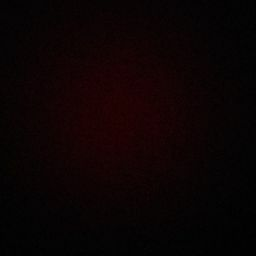# In a simply supported slab, alternate bars are curtailed at

A. $${\frac{1}{4}^{{\text{th}}}}$$ of the span

B. $${\frac{1}{5}^{{\text{th}}}}$$ of the span

C. $${\frac{1}{6}^{{\text{th}}}}$$ of the span

D. $${\frac{1}{7}^{{\text{th}}}}$$ of the span

This Question Belongs to Civil Engineering >> RCC Structures Design

1.The compressive strength of 100 mm cube as compared to 150 mm cube is always ______

2.Can anyone explain it

Related Questions on RCC Structures Design

If the shear stress in a R.C.C. beam is

A. Equal or less than 5 kg/cm2, no shear reinforcement is provided

B. Greater than 4 kg/cm2, but less than 20 kg/cm2, shear reinforcement is provided

C. Greater than 20 kg/cm2, the size of the section is changed

D. All the above